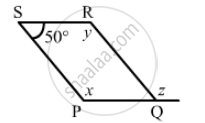Advertisement Remove all ads

# The Following Figure is Parallelogram. Find the Degree Values of the Unknown X, Y, Z. - Mathematics

The following figure is parallelogram. Find the degree values of the unknown xyzAdvertisement Remove all ads

#### Solution

$\text{ Opposite angles of a parallelogram are same } .$
$\therefore x = y \text{ and } \angle RQP = 100°$
$\angle PSR + \angle SRQ = 180°$
$y + 50°= 180°$
$x = 180°- 50°$
$x = 130°$
$\therefore x = 130°, y = 130°$
$\text{ Since y and z are alternate angles, z }= 130° .$

Is there an error in this question or solution?
Advertisement Remove all ads

#### APPEARS IN

RD Sharma Class 8 Maths
Chapter 17 Understanding Shapes-III (Special Types of Quadrilaterals)
Exercise 17.1 | Q 2.2 | Page 9
Advertisement Remove all ads

#### Video TutorialsVIEW ALL 

Advertisement Remove all ads
Share
Notifications

View all notifications

Forgot password?
Course